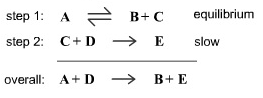# Problem: Consider the following mechanism. Determine the rate law for the overall reaction (where the overall rate constant is represented as k).

###### FREE Expert Solution
97% (316 ratings)
###### FREE Expert Solution

Rate Law represents an equation for a chemical reaction that connects the reaction rate with the concentrations or pressures of the reactants and the rate constant.

Where k is the rate constant

A and B are reactants

x and y are reactant orders

*If the reaction is a single step, the reactant orders can be determined from stoichiometry.

97% (316 ratings)###### Problem Details

Consider the following mechanism.Determine the rate law for the overall reaction (where the overall rate constant is represented as k).